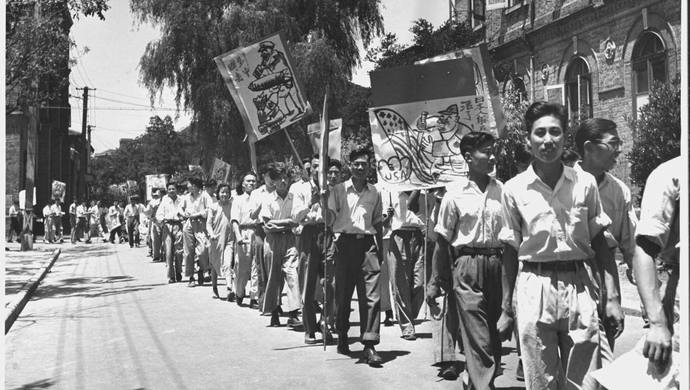5月18日是我参加二野3兵团11军军大的日子。岁月如梭，70年前我义无反顾地选择走向革命道路。七十春秋风雨兼程，尽管国家经历内忧外患，但作为一个受党多年培养教育的老党员来讲，自认为对党的信仰与宗旨坚定不移，也从不敢忘记初心。（责任编辑：士剑波）

• <<<<<<<<<
• <<<<<<<<<
• <<<<<<<<<
• <<<<<<<<<
• <<<<<<<<<
• <<<<<<<<<
• <<<<<<<<<
• <<<<<<<<<
• <<<<<<<<<
• <<<<<<<<<
• <<<<<<<<<
• <<<<<<<<<
• <<<<<<<<<
• <<<<<<<<<
• <<<<<<<<<
• <<<<<<<<<
• <<<<<<<<<
• <<<<<<<<<
• <<<<<<<<<
• <<<<<<<<<
• <<<<<<<<<
• <<<<<<<<<
• <<<<<<<<<
• <<<<<<<<<
• <<<<<<<<<
• <<<<<<<<<
• <<<<<<<<<
• <<<<<<<<<
• <<<<<<<<<
• <<<<<<<<<
• <<<<<<<<<
• <<<<<<<<<
• <<<<<<<<<
• <<<<<<<<<
• <<<<<<<<<
• <<<<<<<<<
• <<<<<<<<<

• <<<<<<<<<

# 商业资讯<<<<<<<<<

• [<<<<<<<<<] <<<<<<<<<
• [<<<<<<<<<] <<<<<<<<<
• [<<<<<<<<<] <<<<<<<<<
• [<<<<<<<<<] <<<<<<<<<
• [<<<<<<<<<] <<<<<<<<<
• [<<<<<<<<<] <<<<<<<<<
• [<<<<<<<<<] <<<<<<<<<
• [<<<<<<<<<] <<<<<<<<<
• [<<<<<<<<<] <<<<<<<<<
• [<<<<<<<<<] <<<<<<<<<
• [<<<<<<<<<] <<<<<<<<<
• [<<<<<<<<<] <<<<<<<<<